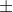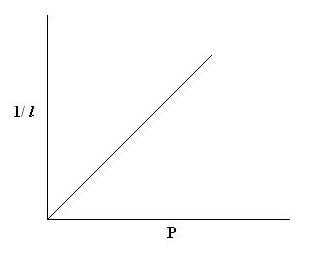you are here->home->Physics->Class 11->Boyle's Law
Boyle's Law

• Quill tube
• Stand
• Metre scale

# Real Lab Procedure

• Arrange the quill tube horizontally on a stand.
• The length of the air column is measured using a metre scale.
• Vertical heights at the two ends of mercury thread from the table are also measured using a metre scale.
• The difference between them gives the vertical height ‘h’ of the mercury thread. Here h=0. So pressure inside the tube is also H, which is the atmospheric pressure.ie; 76 cm of Hg
• The quill tube is then placed in a slanting position with the open end upwards.
• The length of the air column is measured and the vertical height, h of Mercury is noted. Now the pressure inside the tube, P=H + h.
• Quill tube is then placed in different positions, such as: vertical position with open end upwards and with open end downwards, slanting position with open end downwards and measure its corresponding length of the air column l and vertical height h.
• Now P×l  is calculated in each case.

# Simulator Procedure – (As performed through the Online labs)

• Use the ‘Mercury column length (cm)’ slider to change the length of the mercury column.
• Use the ‘Air column length (cm)’ slider to change the length of the air column.
• The atmospheric pressure (H) is already shown in the window.
• We can change the position of the quill tube by dragging it in different directions.
• Click on the ‘Show Scale’ button to see the 50 cm scale for taking measurements.
• We can click the arrows on the scale to rotate the scale.  Also, click and drag the scale to move it to different positions.
• Now, take the readings and calculations are done as per the observation column.
• Click on the ‘Reset’ button to redo the experiment.

# Observations

 Sl No. Position of the tube Height of Hg thread from table to Vertical height, h = h2 - h1  (cm) Pressure,  P = Hh (cm) Length of air column, l (cm) P×l (cm2) Lower end, h1 (cm) Upper end, h2 (cm) 1 Vertical       (open end up) 2 Slanting      (open end up) 3 Horizontal 4 Slanting      (open end down) 5 Vertical       (open end down)

# Calculations

• Calculate P × l in each case.
• Plot a graph between Pressure and reciprocal of length, taking pressure (P) along X axis and reciprocal length (1/l) along Y axis.# Result

From the tabular column, it is found that; P × l is a constant.

The graph between reciprocal of length and pressure is a straight line, which shows that pressure of the given mass of gas is inversely proportional to length of air column.

It indicates the inverse proportionality of pressure and volume of a given mass of gas, hence verifies Boyle’s law.

Cite this Simulator: